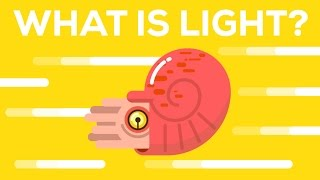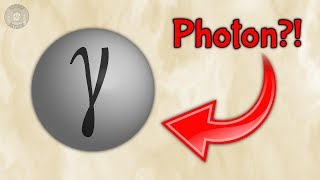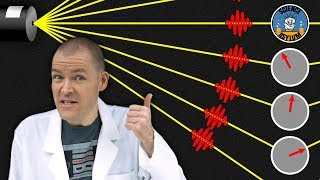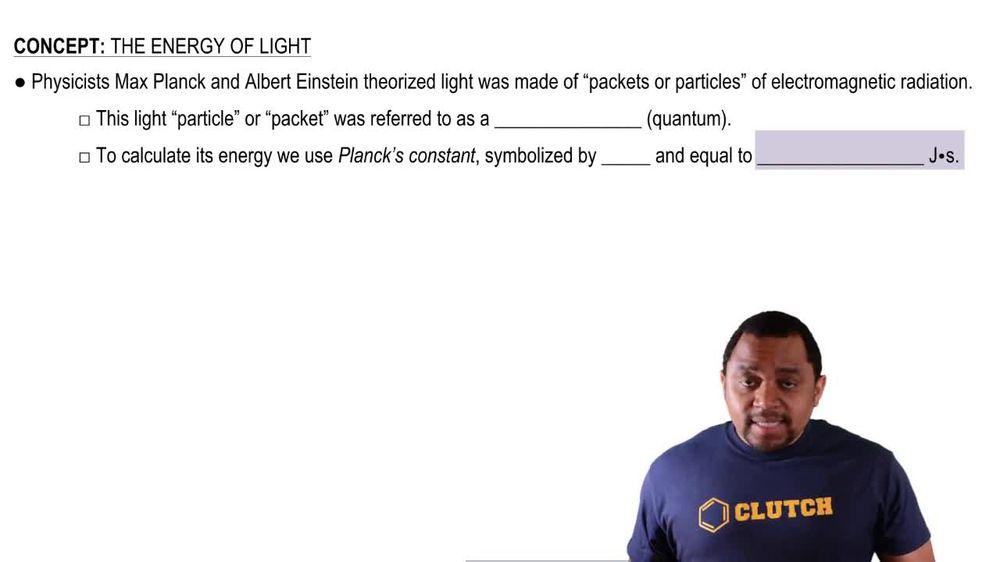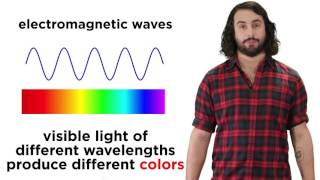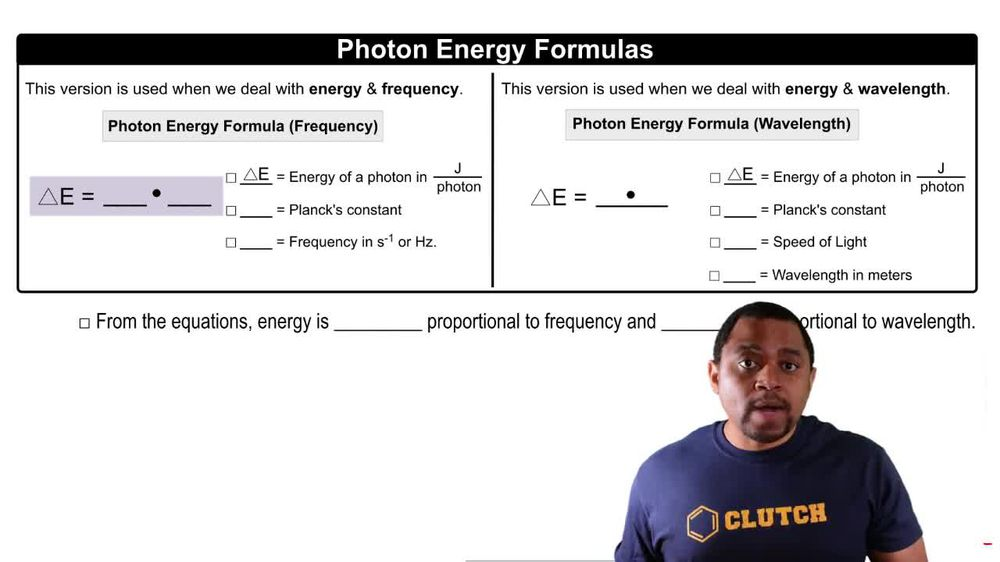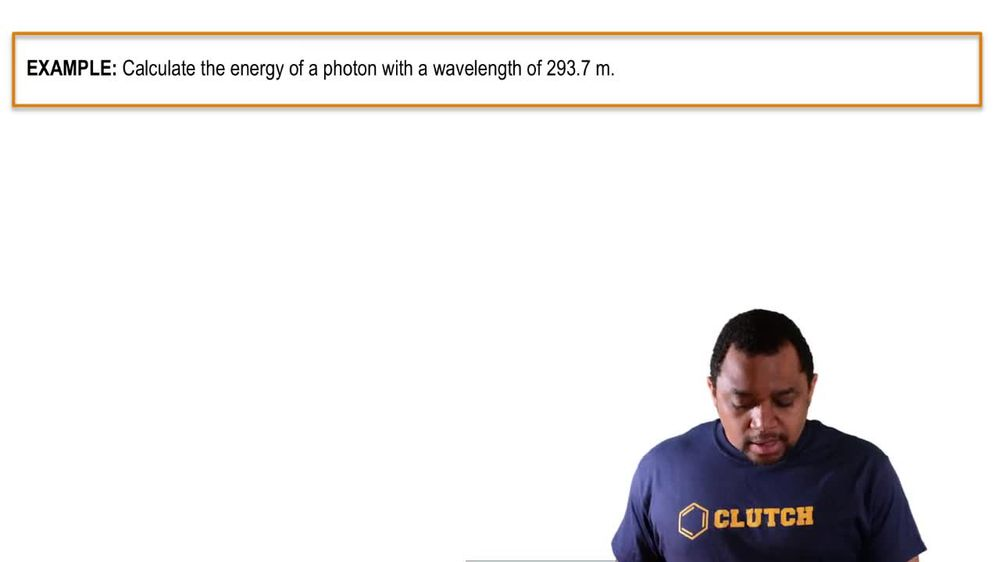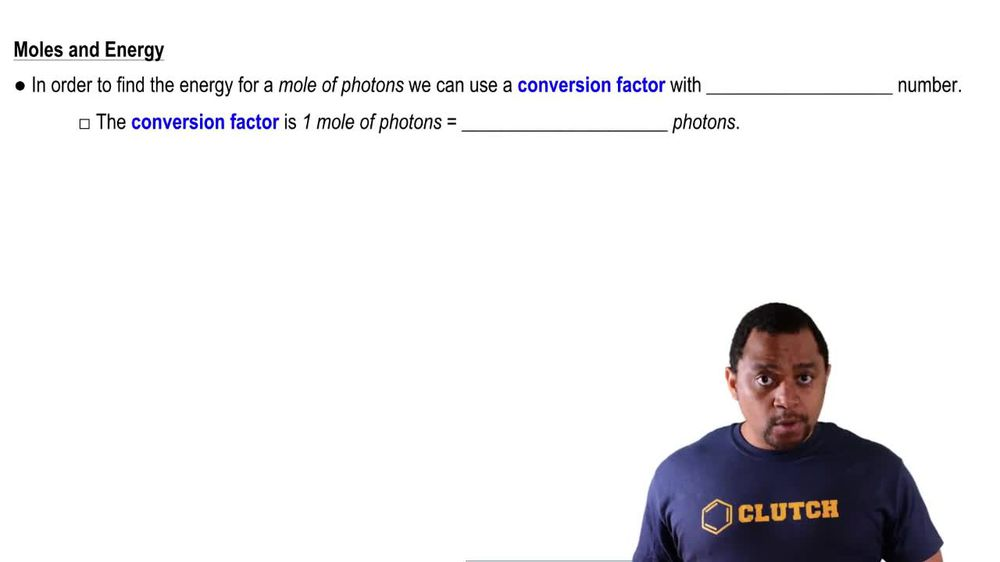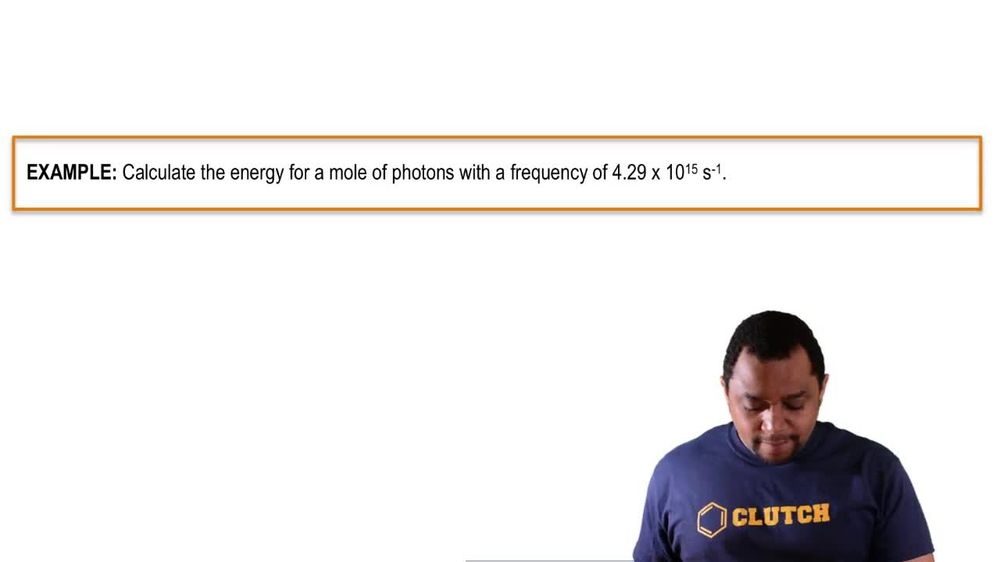Start typing, then use the up and down arrows to select an option from the list.
1. 9. Quantum Mechanics2. The Energy of Light# The Energy of Light Example 1

by Jules Bruno
176 views
1
2
1
here we're told to calculate the energy of a photon with the wavelength of to 93. m. Here they're giving us wavelength off the photon and they're asking for energy. So we're gonna use the second formula. Were the energy of a photon equals plank's constant times the speed of light divided by our wavelength. Here, Plank's constant is 6.626 times 10 to the negative, 34 jewels. Time seconds. Speed of light is 300 times 10 to the 8 m per second. And then we have our wavelength, which is to 93.7 m here. Seconds. Cancel out with seconds meters. Cancel out with meters. That leaves us jewels as our remaining unit. But remember, this is Jules per photon. So here this comes out to 6.768 times 10 to the negative 28 jewels per photon. Here are value has four sig figs because our to 93. m given to us initially also has four significant figures